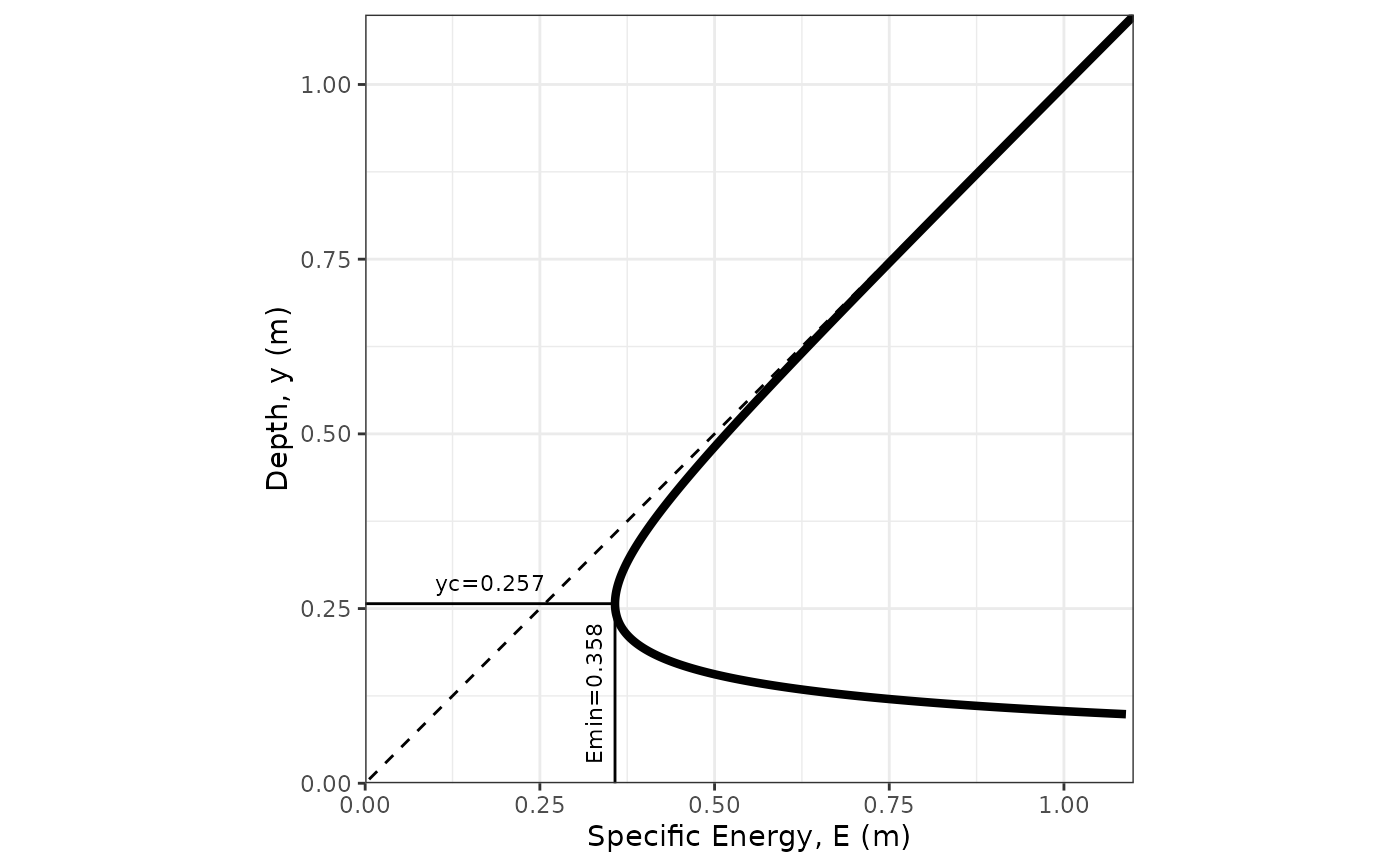This function plots a specific energy diagram of a trapezoidal (including rectangular and triangular) channel, with annotation of critical depth and minimum specific energy.

## Usage

spec_energy_trap(
Q = NULL,
b = NULL,
m = NULL,
y = NULL,
scale = 3,
units = c("SI", "Eng")
)

## Arguments

Q

flow rate [$$m^3 s^{-1}$$ or $$ft^3 s^{-1}$$]

b

bottom width [$$m$$ or $$ft$$]

m

side slope (H:1) [unitless]

y

depth of flow [$$m$$ or $$ft$$] (optional)

scale

multiplier (of yc) for axis scales (default is 3)

units

character vector that contains the system of units [options are SI for International System of Units and Eng for English (US customary) units.

## Value

a specific energy diagram

## Details

Specific Energy, E, is the energy, expressed as a head (i.e., the mechanical energy per unit weight of the water, with units of length) relative to the channel bottom. It is calculated as: $$E = y+\alpha\frac{Q^{2}}{2g\,A^{2}} = y+\alpha\frac{V^{2}}{2g}$$ where $$y$$ is flow depth, $$A$$ is the cross-sectional flow area, $${V}=\frac{Q}{A}$$, and and $$\alpha$$ is a kinetic energy correction factor to account for non-uniform velocities across the cross-section; $$\alpha=1.0$$ in this function (as is commonly assumed).

Ed Maurer

## Examples


# Draw a specific cross-section with flow 1, width 2, side slope 3:1 (H:V)
spec_energy_trap(Q = 1.0, b = 2.0, m = 3.0, scale = 4, units = "SI")